# MA.912.GR.7.2Export Print
Given a mathematical or real-world context, derive and create the equation of a circle using key features.

### Clarifications

Clarification 1: Instruction includes using the Pythagorean Theorem and completing the square.

Clarification 2: Within the Geometry course, key features are limited to the radius, diameter and the center.

General Information
Subject Area: Mathematics (B.E.S.T.)
Strand: Geometric Reasoning
Status: State Board Approved

## Benchmark Instructional Guide

• Circle
• Diameter

### Vertical Alignment

Previous Benchmarks

Next Benchmarks

### Purpose and Instructional Strategies

In grade 8, students solved problems involving the Pythagorean Theorem. In Algebra 1, students rearranged formulas to highlight a quantity of interest, worked with quadratic equations and functions and performed single transformations on functions. In Geometry, this background provides a solid foundation for writing the equation of a circle to represent a variety of contexts, based on key features of the circle. In later courses, students will expand these skills to write equations for other circles, parabolas, hyperbolas and ellipses based on their key features.
• Instruction includes students deriving the equation of a circle centered at the origin and at a point ($h$, $k$). Students should make the connection to the Pythagorean Theorem by drawing right triangles from the center of the circle. Students should make the connection between the equations centered at the origin and at a point ($h$, $k$); realizing that if the point ($h$, $k$) is the origin then the equation can be written as either $x$2 + $y$2 = $r$2 or as ($x$ − 0)2 + ($y$ − 0)2 = $r$2
• For example, given a circle centered at the origin on the coordinate plane and have students select a point $P$($x$, $y$) on the circle. Students should be able to see that the distance from $P$ to the center is the radius of the circle. Students can then draw a right triangle, like the one shown below, that includes the center and $P$ as two of its vertices. Students can use their knowledge of the Pythagorean Theorem to develop the equation $x$2 + $y$2 = $r$2, showing the relationship between $x$, $y$ and the radius, $r$. Students should be able to realize that this equation is valid for every point P($x$, $y$) on the circle and is not valid for any point not on the circle.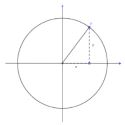• For example, given a circle centered at $C$($h$, $k$) on the coordinate plane and have students select a point $P$($x$, $y$) on the circle. Students should be able to see that the distance from $P$ to the center is the radius of the circle. Students can then draw a right triangle, like the one shown below, that includes the center and $P$ as two of its vertices. Students can use their knowledge of the Pythagorean Theorem to develop the equation ($x$$h$)2 + ($y$$k$)2 = $r$2, showing the relationship between $x$, $y$, $h$, $k$ and the radius, $r$. Students should be able to realize that this equation is valid for every point $P$($x$, $y$) on the circle and is not valid for any point not on the circle.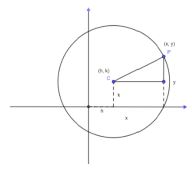• Instruction includes the connection to transformation of functions (as was done in Algebra 1) when developing an understanding of the relationship between the equations $x$2 + $y$2 = $r$2 and ($x$$h$)2 + ($y$$k$)2 = $r$2
• Students should have experience working with the equation of a circle in center-radius form, ($x$$h$)2 + ($y$$k$)2 = $r$2, and in general form, $A$$x$2 + $B$$x$2 + $C$$x$ + $D$$y$ + $E$ = 0 where $A$ and $B$ are the same real, non-zero number. Students may need to convert from one form to another, particularly from the general form to the center-radius form. Instruction includes discussing how this conversion can be done and the benefits of each form of the equation of a circle.
• Instruction includes exploring the pattern of completing the square for the general form and the way the center and the radius can be obtained from this procedure. Exploration includes cases where the radius may result in a complex number; noting that the equation does not give a circle when this happens.
• For example, given the equation $A$$x$2 + $B$$y$2 + $C$$x$ + $D$$y$ + $E$ = 0 when $A$ = 1 and $B$ = 1, students can rewrite this as $x$2 + $C$$x$ + $y$2 + $D$$y$ = −$E$. Then students can complete the square for both the $x$-part of the equation and the $y$-part of the equation. Students should obtain the equation ($x$ + $\frac{\text{C}}{\text{2}}$)2 + ($y$ + $\frac{\text{D}}{\text{2}}$)2 = −$E$ + ($\frac{\text{C}}{\text{2}}$)2 + ($\frac{\text{E}}{\text{2}}$)2. Students should then compare this to center-radius form to determine that the center of the circle is at the point (−$\frac{\text{C}}{\text{2}}$, −$\frac{\text{D}}{\text{2}}$) and the radius is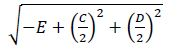• Problem types include cases where $A$ and $B$ are equivalent, but not equal to 1.
• For enrichment of this benchmark, instruction includes the connection to finding the equation of the circumscribed circle about a triangle whose vertices are given on the coordinate plane. (MTR.5.1)

### Common Misconceptions or Errors

• Students may confuse the signs of ($h$$k$) when determining the center of the circle.
• For example, given the equation ($x$ − 1)2 + ($y$ + 2)2 = 1, the center is (1, −2) and not (−1, 2). • Students may forget to take the square root of the $r$2 term to determine the radius of the circle.

• A circle on the coordinate plane is given. Segments $C$$B$ and $E$$D$ are diameters of circle $A$. Point $C$ is located at (3,5), point $D$ is located at (6,6), point $B$ is located at (7,3) and point $E$ is located at (4,2).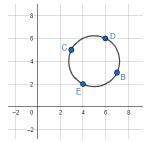• Part A. Determine the center of the circle. Explain your method.
• Part B. Find the length of the radius of circle $A$. Explain your method.
• Part C. Write the equation of the circle and check that the points $B$, $C$, $D$ and $E$ satisfy the equation.

• Point ($x$, $y$) is on a circle with center ($h$, $k$).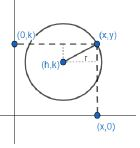• Part A. The horizontal distance between point ($x$, $y$) and center ($h$, $k$) can be represented as _____. The vertical distance between the point ($x$, $y$) and center ($h$, $k$) can be represented as _____.
• Part B. Using Pythagorean Theorem, write an equation for the radius in terms of ($x$, $y$) and ($h$, $k$).
• Part C. Because ($x$, $y$) could be any point on the circle, this is the equation of a circle where ($h$, $k$) is the ______________ of the circle and r is the _____________ of the circle.

• A school’s campus is designed in the shape of a circle. The architect would like to place the cafeteria equidistant from the Freshman building and from the Senior building. On a coordinate plane, the Freshman building is located at the point (431, 219) and the Senior building is located at the point (0,0), where the coordinates are given in feet. Assume that the endpoints of the diameter of circle are the Freshman building and the Senior building and that the cafeteria is on the line connecting the two buildings.
• Part A. Determine the location of the cafeteria.
• Part B. How far is it from the cafeteria to the Freshman building?
• Part C. Write an equation that represents the boundary of the circular campus.
• Part D. If the campus were to have a circular fence along its boundary, what is the total length of the fence, in feet?

### Instructional Items

Instructional Item 1
• Given the equation $x$2 + 2$x$ + $y$2 − 4$y$ + $E$ = 0, determine possible values of $E$ such that the equation is an equation of a circle.

Instructional Item 2
• What is the equation of a circle centered at (−1, 2), with a diameter of 2 units?

Instructional Item 3
• What is the equation of the circle centered at (−2, −5) and passing through (5, 0)?

*The strategies, tasks and items included in the B1G-M are examples and should not be considered comprehensive.

## Related Courses

This benchmark is part of these courses.
1200400: Foundational Skills in Mathematics 9-12 (Specifically in versions: 2014 - 2015, 2015 - 2022, 2022 and beyond (current))
1202340: Precalculus Honors (Specifically in versions: 2014 - 2015, 2015 - 2022, 2022 and beyond (current))
1206310: Geometry (Specifically in versions: 2014 - 2015, 2015 - 2022, 2022 and beyond (current))
1206320: Geometry Honors (Specifically in versions: 2014 - 2015, 2015 - 2022, 2022 and beyond (current))
1206315: Geometry for Credit Recovery (Specifically in versions: 2014 - 2015, 2015 - 2022, 2022 and beyond (current))
7912065: Access Geometry (Specifically in versions: 2015 - 2022, 2022 and beyond (current))

## Related Access Points

Alternate version of this benchmark for students with significant cognitive disabilities.
MA.912.GR.7.AP.2: Create the equation of a circle when given the center and radius.

## Related Resources

Vetted resources educators can use to teach the concepts and skills in this benchmark.

## Formative Assessments

Complete the Square for Center-Radius 2:

Students are asked to find the center and radius of a circle given its equation in general form.

Type: Formative Assessment

Derive the Circle – General Points:

Students are given the coordinates of the center, (h, k), and the radius, r, of a circle and are asked to derive the equation of the circle using the Pythagorean Theorem.

Type: Formative Assessment

Derive the Circle – Specific Points:

Students are given the coordinates of the center and the radius of a circle and are asked to derive the equation of the circle using the Pythagorean Theorem.

Type: Formative Assessment

Students are asked to find the center and radius of a circle given its equation in general form.

Type: Formative Assessment

## Lesson Plans

Circle Reasoning:

Students use the Pythagorean Theorem (Distance Formula) to derive the Standard Equation of a Circle; then move between descriptions and equations of a circle.

Type: Lesson Plan

Run Fido, Run!:

A guided practice for deriving the equation of a circle and then identifying a location to tether a dog to maximize movement.

Type: Lesson Plan

## Original Student Tutorials

Where IS that Cell Tower?:

Find the location and coverage area of cell towers to determine the center and radius of a circle given its equation, using a strategy completing the square in this interactive tutorial.

Type: Original Student Tutorial

Circle Up!:

Learn how to write the equation of a circle using Pythagorean Theorem given its center and radius using step-by-step instructions in this interactive tutorial.

Type: Original Student Tutorial

## MFAS Formative Assessments

Students are asked to find the center and radius of a circle given its equation in general form.

Complete the Square for Center-Radius 2:

Students are asked to find the center and radius of a circle given its equation in general form.

Derive the Circle – General Points:

Students are given the coordinates of the center, (h, k), and the radius, r, of a circle and are asked to derive the equation of the circle using the Pythagorean Theorem.

Derive the Circle – Specific Points:

Students are given the coordinates of the center and the radius of a circle and are asked to derive the equation of the circle using the Pythagorean Theorem.

## Original Student Tutorials Mathematics - Grades 9-12

Circle Up!:

Learn how to write the equation of a circle using Pythagorean Theorem given its center and radius using step-by-step instructions in this interactive tutorial.

Where IS that Cell Tower?:

Find the location and coverage area of cell towers to determine the center and radius of a circle given its equation, using a strategy completing the square in this interactive tutorial.

## Student Resources

Vetted resources students can use to learn the concepts and skills in this benchmark.

## Original Student Tutorials

Where IS that Cell Tower?:

Find the location and coverage area of cell towers to determine the center and radius of a circle given its equation, using a strategy completing the square in this interactive tutorial.

Type: Original Student Tutorial

Circle Up!:

Learn how to write the equation of a circle using Pythagorean Theorem given its center and radius using step-by-step instructions in this interactive tutorial.

Type: Original Student Tutorial

## Parent Resources

Vetted resources caregivers can use to help students learn the concepts and skills in this benchmark.